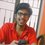# Ashish's $\LaTeX$ playground

$\large \text {This is my note for testing} \LaTeX$ $:P$

$\large \text {A special thanks to}$ $\large \color{#D61F06}{\text {Pi Han Goh}}$ $\large \text {and}$ $\large \color{#3D99F6}{\text {Andrew Ellinor}}$ $\large \text {and}$ $\large \color{#20A900}{\text {Nihar Mahajan}}$$\large \text {for the success of this note.}$ \begin{aligned} \begin{array}{c}x & = 2+5 \\ & = 7 \end {array} \end{aligned}

$\begin {pmatrix} 1 & & 0\\ 0 & & 1\ \end {pmatrix}$ × $\begin {pmatrix} 1 & & 0\\ 0 & & 1\ \end {pmatrix}$ = $\begin {pmatrix} 1+0 & & 0+0\\ 0+0 & & 0+1\ \end {pmatrix}$ = $\begin {pmatrix} 1 & & 0\\ 0 & & 1\ \end {pmatrix}$

$\large{\color{magenta}{\text{My name is Ashish}}}$

\begin{aligned} & x + 7x & = 24\\ \implies & 8x & =24\\ \implies & x & = \dfrac {24}{8}\\ \therefore & x & = \boxed{3} \end{aligned}

$\ce {{Na}_2CO_3 + H_2O -> NaOH + H_2O + CO_2}$

$\begin{pmatrix} \nearrow \circ \nwarrow & \approx & \nearrow \circ \nwarrow\\ & \bigcup & \\ & \nearrow \searrow \nearrow \searrow \nearrow \searrow & \\& \smile & \end{pmatrix}$

$\LARGE{ \begin{array}{rll} \phantom{0}\ \boxed{{5}} && \-2pt] \boxed{{5}}\ \enclose{longdiv}{\boxed{{3}} \ \boxed{7}}\kern-.2ex \\[-2pt] \underline{\boxed{{3}} \ \boxed{{5}}} && \\[-2pt] \boxed{{2}} \end{array} }$ $\LARGE{ \begin{array}{rll} \boxed{{x}}\ + \boxed{{1}} && \\[-2pt] \boxed{{x}}\ + \boxed{{1}} \enclose{longdiv}{\boxed{{x^2}}\ + \boxed{{2x}}\ + \boxed{{1}}}\kern-.2ex \\[-2pt] \underline{\boxed{{x^2}}\ + \boxed{{x}}\ \phantom{0}\ \phantom{0}\ \phantom{0}} \phantom{0}\\[-2pt] \phantom{0}\ \boxed{{x}}\ + \boxed{{1}}\kern-.2ex \\[-2pt] \underline{\boxed{{x}}\ + \boxed{{1}}} && \\[-2pt] \phantom{0}\ \phantom{0}\ \boxed{{0}} \end{array} }$ \begin{aligned} \begin {array} x & \dfrac{29 \times 7}{116}\\ = & \dfrac{{{\cancel {29}}} \times 7}{{\require {cancel}{\cancel {116}}} 4}\\ = & \boxed {\dfrac{7}{4}} \end {array} \end{aligned} $\huge \text{I ♡ Brilliant}$ $\large \text{Here are some symbols} :- ♤■□●◇◆•○♡♧¡¿《》¤°♧▪$ \begin{aligned} \dfrac {10}{20} & =\require {cancel}{\dfrac {\xcancel{10}}{\xcancel{20}2}}\\ & = \dfrac {1}{2} \end{aligned} ${\Huge {\color{#D61F06}{B}}}{\huge{\color{#3D99F6}{R}}}{\LARGE {\color{#CEBB00}{I}}}{\Large{\color{#20A900}{L}}}{\large{\color{magenta}{L}}}{\Large{\color{#69047E}{I}}}{\LARGE{\color{#333333}{A}}}{\huge{\color{#E81990}{N}}}{\Huge{\color{#624F41}{T}}}$ \begin{aligned} 5+4 & = 8 +1\\ & = 9 \\ \\ 7+2 & = 8+1\\ & = 9 \end{aligned} $\Huge \sqrt[\sqrt[x^5]{\sqrt[x^4]{\sqrt[x^3]{\sqrt[x^2]{\sqrt[x]{x}}}}}]{\sqrt[5\sqrt[x]{\sqrt[2x]{\sqrt[3x]{\sqrt[4x]{x}}}}]{\sqrt[5x]{\sqrt[4x]{\sqrt[3x]{\sqrt[2x]{x}}}}}} = x^{x^{5 + \tfrac{x^{11} + 24}{24x^{15}}}}$ Find the real value of $x$ satisfying the real equation above. $\text{Note}$:- Here $x \neq \{-1 , 0 , 1\}$ $\sqrt[\sqrt{{\left(\sqrt[x]{4x^4}\right)}^{x^4}}]{\sqrt{\sqrt[x]{4}}}$ = $4^{-17x^{-x^3 - 1}}$ $\sqrt{{\left(\sqrt[x]{4x^4}\right)}^{x^4}}$ ${\sqrt{\sqrt[x]{4}}}^{\tfrac{1}{\sqrt{{\left(\sqrt[x]{4x^4}\right)}^{x^4}}}}$ $\log_8 \log_4 \color{#3D99F6}{\log_2 16}\\ = \log_8 \log_4 \color{#3D99F6}{4}\\ = \log_8 \color{#D61F06}{\log_4 4}\\ = \log_8 \color{#D61F06}{1}\\ = \color{#20A900}{\log_8 1}\\ = \color{#69047E}{\boxed{0}}$ $\log_{\color{#3D99F6}{b}} \color{#D61F06}{a} = \dfrac{\log \color{#D61F06}{a}}{\log \color{#3D99F6}{b}}$ $\log_{\color{#3D99F6}{\sqrt{2}}} \color{#D61F06}{m}\\ \\ = \dfrac{\log \color{#D61F06}{m}}{\log \color{#3D99F6}{2^{\tfrac{1}{2}}}}\\ \\ = \dfrac{\log m}{\log 2^{\color{#20A900}{\tfrac{1}{2}}}}\\ \\ = \dfrac{\log m}{\color{#20A900}{\dfrac{1}{2}} \log 2}\\ \\ = \dfrac{1}{\frac{1}{2}} \dfrac{\log \color{cyan}{m}}{\log \color{magenta}{2}}\\ \\ = \boxed{2} \log_{\color{magenta}{2}} \color{cyan}{m}$Note by Ashish Menon 5 years, 1 month ago This discussion board is a place to discuss our Daily Challenges and the math and science related to those challenges. Explanations are more than just a solution — they should explain the steps and thinking strategies that you used to obtain the solution. Comments should further the discussion of math and science. When posting on Brilliant: • Use the emojis to react to an explanation, whether you're congratulating a job well done , or just really confused . • Ask specific questions about the challenge or the steps in somebody's explanation. Well-posed questions can add a lot to the discussion, but posting "I don't understand!" doesn't help anyone. • Try to contribute something new to the discussion, whether it is an extension, generalization or other idea related to the challenge. • Stay on topic — we're all here to learn more about math and science, not to hear about your favorite get-rich-quick scheme or current world events. MarkdownAppears as *italics* or _italics_ italics **bold** or __bold__ bold - bulleted- list • bulleted • list 1. numbered2. list 1. numbered 2. list Note: you must add a full line of space before and after lists for them to show up correctly paragraph 1paragraph 2 paragraph 1 paragraph 2 [example link](https://brilliant.org)example link > This is a quote This is a quote  # I indented these lines # 4 spaces, and now they show # up as a code block. print "hello world" # I indented these lines # 4 spaces, and now they show # up as a code block. print "hello world" MathAppears as Remember to wrap math in $$ ... $$ or \[ ...  to ensure proper formatting.
2 \times 3 $2 \times 3$
2^{34} $2^{34}$
a_{i-1} $a_{i-1}$
\frac{2}{3} $\frac{2}{3}$
\sqrt{2} $\sqrt{2}$
\sum_{i=1}^3 $\sum_{i=1}^3$
\sin \theta $\sin \theta$
\boxed{123} $\boxed{123}$

Sort by:

Any suggestions? I am practicing this for mastering LaTeX especially long division of polynomials.

- 5 years, 1 month ago

- 5 years, 1 month ago

Thank you, accept my THANKS, ;P

- 5 years, 1 month ago

Thank u sir. Im a beginner now I can learn latex.

- 4 years, 12 months ago

THANK YOU Ashish for creating this note ..helped me to learn latex..thanks a ton.!!:)

- 4 years, 12 months ago

Entirely welcome

- 4 years, 12 months ago